# Electrical resistance facts for kids

Kids Encyclopedia Facts

The electrical resistance of an electrical conductor is a measure of the difficulty of passing an electric current through that conductor. It explains the relationship between voltage (amount of electrical pressure) and the current (flow of electricity). With more resistance in a circuit, less electricity will flow through the circuit.

Resistance, discovered by Georg Simon Ohm in 1827, is the ratio between voltage and current. Ohm's law said that the voltage between any two points in a conductor changes directly as the current between the two points, given the temperature remains the same. He described it with the equation: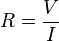$R = \frac{V}{I}$

which models the ratio, where: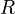${\textstyle R}$ is the resistance of the object, measured in ohms (Ω)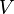${\textstyle V}$ is the voltage across the object, measured in volts (V)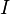${\textstyle I}$ is the current going through the object, measured in amperes (A)

## Calculating resistance

The resistance of a wire increases as it becomes longer and decreases as it becomes wider. (A simple analogy is a road - the more lanes there are, the less traffic there is.) The resistance R of a wire with a constant width, therefore, can be calculated as: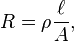$R = \rho\frac{\ell}{A}, \,$

where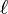$\ell$ is the length of the conductor, measured in meters [m],${\textstyle A}$ is the cross-sectional area of the conductor measured in square meters [m²], and ρ (Greek: rho) is the electrical resistivity (also called specific electrical resistance) of the material, measured in ohm-meters (Ω m).

Example: Calculate the resistance of copper wire with a radius of 2mm and a length of 5 meters.

Solution:

The resistivity (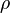$\rho\,$) of copper is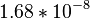$1.68*10^{-8} \,$ Ω m.
The cross sectional area ($A\,$) is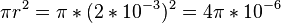$\pi r^2=\pi *(2*10^{-3})^2=4\pi *10^{-6}\,$ square meters
The length (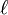$\ell\,$) is$5\,$ meters

Because:$R = \rho\frac{\ell}{A}, \,$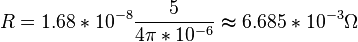$R = 1.68*10^{-8} \frac{5}{4\pi *10^{-6}}\thickapprox 6.685*10^{-3}\Omega \,$

## Applications

Resistors are used in electrical circuits to provide electrical resistance.

## Images for kidsElectrical resistance Facts for Kids. Kiddle Encyclopedia.